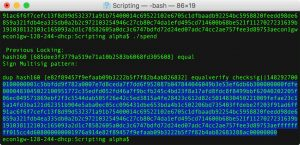# Libbitcoin Multi-Signature: Making a Payment

Spending from a miltisig address isn’t a super complex procedure but is prone to mistakes, so its best to practice on the test net.

This program creates a raw transaction from a testnet 2-of-3 multisig address. Creating a multisig transaction is similar to creating a standard transaction except for the signing process which will allow me to create functions out of the routines I used in my rawTX program. So I’m going to need to:

• Build the input
• from utxo
• Build the the output
• Build the transaction
• Sign The transaction
• Build and Add The signature script to the Transaction
• Output the raw transaction.
```

#include <bitcoin/bitcoin.hpp>
#include "HD_walletTESTNET.cpp"
#include <string.h>

using namespace bc;
using namespace bc::wallet;
using namespace bc::machine;
using namespace bc::chain;

```

So, I’m going to need to first create a function to deal with the unspent transaction output, here I convert the string of a transaction ID to an output point at index 0. I do this by using libbitcoin’s decode hash function on the txid string and passing the hash along with the utxo’s index to an output point constructor.

```
//previous output
output_point getUTXO()
{
hash_digest previousTX;
decode_hash(previousTX, "");
return output_point(previousTX, 0u);
}

```

Now, I can use the utxo object to create the transactions input using the libbitcoin input setters. I also nullify the transaction sequence field by passing 0xffffffff.

```
//input
input makeInput()
{
input input1 = input();
output_point utxo = getUTXO();
input1.set_previous_output(utxo);
input1.set_sequence(0xffffffff);
return input1;
}

```

Now that I have a way to return the transactions input I need to create the output. So, I’ll use the destination address and a script stack factory to return the locking script. After that I can create the output using the script and the amount in satoshis.

```
//output
script makelockingScript()
{
return (script().to_pay_key_hash_pattern(destination.hash()));
}
output makeOutput()
{
uint64_t amount = 148292700;
script lockingScript = makelockingScript();
return output(amount, lockingScript);
}

```

Now I can use these functions to create my transaction by simply pushing the input and output to the transaction object’s list member.

```
//transaction object
transaction makeTX()
{

transaction tx = transaction();
input input1 = makeInput();
output output1 = makeOutput();
tx.inputs().push_back(input1);
tx.outputs().push_back(output1);
return tx;
}
```

Once we have out transaction, we need to sign it with two out of the three wallets. In order to create the signature I’ll need to sign the transaction object and the redeem script that created the multsig wallet. The redeem script needs to be constructed in it’s original order of public keys.

I’ve used the following function to create the redeem script out of 3 public key data chunks using a stack factory.

```
//Redeem Script
script redeemScript(data_chunk p1, data_chunk p2, data_chunk p3)
{
data_stack keys {p1, p2, p3};
script multisigScript = script(script().to_pay_multisig_pattern(2, keys));
return multisigScript;

}
```

Now we can sign all the parts of the transaction by passing them with an HD_Wallet object and using a script endorsement function. This function is going to assume I’m signing with the wallets first child.

```
//signing
endorsement signature(data_chunk p1, data_chunk p2, data_chunk p3, transaction tx, HD_Wallet wallet1)
{
endorsement endorsed;
if(script().create_endorsement(endorsed, wallet1.childPrivateKey(1).secret(), redeemScript(p1, p2, p3), tx, 0, all))
{
return endorsed;
} else {
std::cout << "Signature Failed!! " << std::endl;
return endorsed;
}
}
```

The signing function can be used for both signatures, which means I now just need to write a function that builds the final signature script and I can put it all together in the main function. Here, I build the final script out of operations passing OP_0 (because of a bug in the scripting language), the two signatures and the serialized redeem script.

```
script buildSigScript(endorsement endorsed1, endorsement endorsed2, data_chunk p1, data_chunk p2, data_chunk p3)
{
script redeem = redeemScript(p1, p2, p3);

operation::list ops = {operation(opcode(0)), operation(endorsed1), operation(endorsed2), operation(redeem.to_data(0))};

if(script().is_sign_multisig_pattern(ops))
{
std::cout<< "Sign Multisig pattern: " << std::endl;
return script(ops);
}else{
std::cout << "Not sign Multisig pattern: " << std::endl;
return script(ops);
}
}
```

Now I can build the entire raw transaction in the main function. Starting by importing the wallets to use by their mnemonic seeds. In reality, I wouldn’t have access to the other wallet so I’d need to have the redeem script saved and pass my signature to my co-signer. However, for this purpose I’ll just bring in all three so I can rebuild the redeem script from their public keys.

```
//Display

int main()
{
std::string Mnemonic1 = "";
std::string Mnemonic2 = "";
std::string Mnemonic3 = "";

HD_Wallet wallet1(split(Mnemonic1));
HD_Wallet wallet2(split(Mnemonic2));
HD_Wallet wallet3(split(Mnemonic3));

data_chunk p1 = to_chunk(wallet1.childPublicKey(1).point());
data_chunk p2 = to_chunk(wallet2.childPublicKey(1).point());
data_chunk p3 = to_chunk(wallet3.childPublicKey(1).point());
```

Then I can just make the transaction.

```
transaction tx = makeTX();
tx.set_version(1);
```

And now generate both signatures before building the signature script and adding it to the transaction via the set_script member of the input.

```
endorsement sig1 = signature(p1, p2, p3, tx, wallet1);
endorsement sig2 = signature(p1, p2, p3, tx, wallet2);
script sigScript = buildSigScript(sig1, sig2, p1, p2, p3);
tx.inputs().set_script(sigScript);
```

Now, The transaction is fully formed and signed. We can output a summary of the transaction by accessing the first output’s script and value accessors. After that, we can base16 encode the raw transaction and output it to the user.  This raw transaction can be pushed to testnet via blockcypher or bx.

```
std::cout << "\n" <<tx.outputs().script().to_string(0xffffffff) << "||" << tx.outputs().value() << std::endl;
std::cout << encode_base16(tx.to_data(1)) << std::endl;
}
```

Script can be run as usual:

```\$ g++ -std=c++11 -o spend multiSpend.cpp \$(pkg-config --cflags libbitcoin --libs libbitcoin)
\$ ./spend
```

And here is the output with the raw transaction highlighted.!